# MP Board Class 6th Maths Solutions Chapter 5 Understanding Elementary Shapes Ex 5.8

## MP Board Class 6th Maths Solutions Chapter 5 Understanding Elementary Shapes Ex 5.8

Question 1.
Examine whether the following are polygons. If any one among them is not, say why?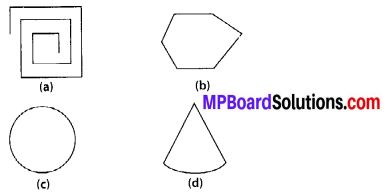Solution:
(a) As it is not a closed figure, therefore, it is not a polygon.
(b) It is a polygon because it is closed by line segments.
(c) It is not a polygon because it is not made by line segments.
(d) It is not a polygon because it is not made by only line segments and also it has curved surface.Question 2.
Name each polygon.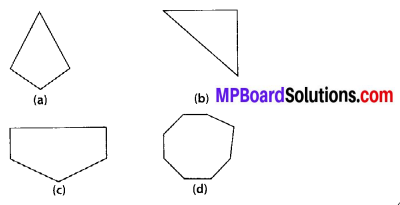Make two more examples of each of these.
Solution:
(b) Triangle
(c) Pentagon
(d) Octagon
Two more examples of each :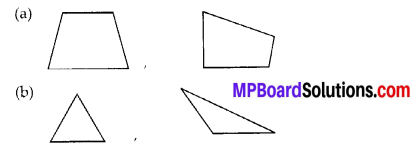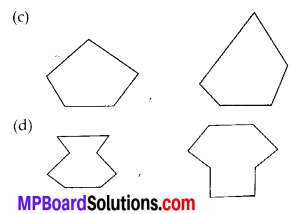Question 3.
Draw a rough sketch of a regular hexagon. Connecting any three of its vertices, draw a triangle. Identify the type of the triangle you have drawn.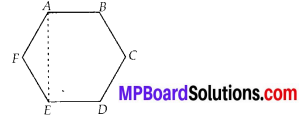Solution:
ABCDEF is a regular hexagon and ∆AEF is a triangle formed by joining AE.
Hence, ∆AEF is an isosceles triangle.

Question 4.
Draw a rough sketch of a regular octagon. (Use squared paper if you wish). Draw a rectangle by joining exactly four of the vertices of the octagon.
Solution: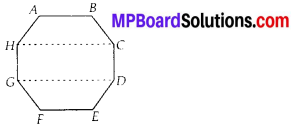ABCDEFGH is a regular octagon and CDGH is a rectangle formed by joining C and H; D and G.Question 5.
A diagonal is a line segment that joins any two vertices of the polygon and is not a side of the polygon. Draw a rough sketch of a pentagon and draw its diagonals.
Solution: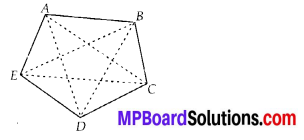ABCDE is the required pentagon and its diagonals are AD, AC, BE, BD and CE.